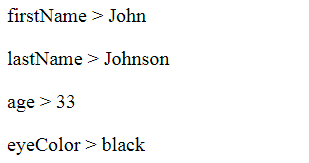# JavaScript Loop: Iterate through an Array or an Object (multiple examples)The JavaScript Loop is used to iterate through an array of items (which can be a number array, string array, etc) or objects. There are many ways to do it and so in this tutorial we will look on them one by one.

Here I have taken an array of numbers and I will do the JavaScript Loop through array by using the different looping methods.

The number array is:

```var numbers = [1, 2, 3, 4, 5];
```

## 1. JavaScript For Loop

In JavaScript For Loop, the iteration is done from 0 to the ‘one less than the length of the array’. That is the loop will execute until the condition is true.

```for (var i = 0; i < numbers.length; i++) {
}
```

The above code will show us each of these 5 numbers in an alert box.

I used a looping method to extract all the values from an XML file. This is explained at this tutorial – Read XML file with jQuery Load Method and display it in a HTML Table

### JavaScript Loop through Object

Now let’s use the JavaScript For Loop to iterate and extract values from an object:

```var student = {
firstName : "John",
lastName  : "Johnson",
age       : 33,
eyeColor  : "black"
};

for (var k in student) {
console.log(k + " > " + student[k]);
}
```

Here I have an object called ‘student’, and it holds some values like first name, last name, age and eye color of a student. I iterated through the student’s info – for (var k in student) and extracted them like student[k].

The output you get is shown by the below image:If you want to quickly create a Modal Popup without Bootstrap then see jQuery Modal PopUp Window with Animation Effects

### JavaScript For Loop Break

The break statement can also be used to jump out of a for loop in JavaScript. In the above example, I introduce the break statement as shown below:

```var numbers = [1, 2, 3, 4, 5];
for (var i = 0; i < numbers.length; i++) {
if (i === 1) { break; }
}
```

I will get only ‘1 alert message’ (instead of 5) because when ‘i’ value becomes 1 then the break statement jumps out of the for loop.

## 2. JavaScript For In Loop

In this loop I use the in keyword with for.

```for (var index in numbers) {
}
```

Note: I have kept the array after the in keyword. The index variable will contain the current index of the loop, so numbers[index] will provide the current value of the array in the loop.

## 3. JavaScript While Loop

The JavaScript while loop iterates through a block of code as long as a specified condition is true.

A simple example for this is given below:

```var i = 0;
while (i < numbers.length) {
i++;
}
```

## 4. JavaScript Do While Loop

The JavaScript Do While loop is similar to the While loop except that this loop will always be executed at least once, even if the condition is false. The reason being the code block is executed before the condition is tested.

```var i = 0;
do {
i++;
}
while (i < 5);
```

## 5. jQuery Each Loop

jQuery Loop is based on jQuery Each method. It is very powerful and amazing method.

The jQuery Loop example is given below:

```\$.each(numbers, function (index, value) {